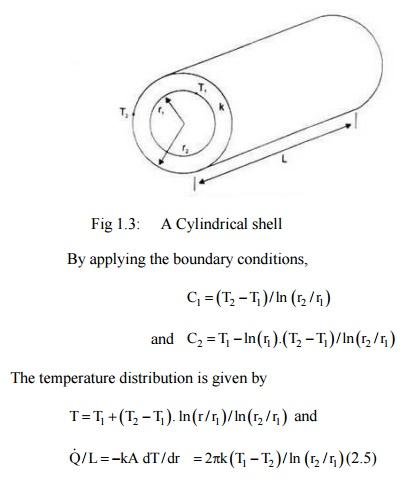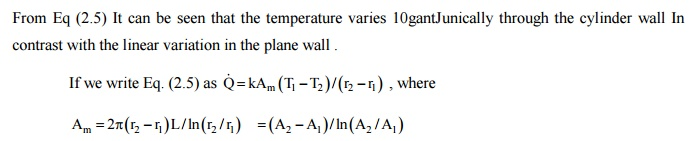Home | | Heat and Mass Transfer | One Dimensional Steady State Equation Plane Wall

# One Dimensional Steady State Equation Plane Wall

The term 'one-dimensional' is applied to heat conduction problem when: (i) Only one space coordinate is required to describe the temperature distribution within a heat conducting body;

ONE DIMENSIONAL STEADY STATE EQUATION PLANE WALL :

The term 'one-dimensional' is applied to heat conduction problem when:

(i)             Only one space coordinate is required to describe the temperature distribution within a heat conducting body;

(ii)           Edge effects are neglected;

(iii)        The flow of heat energy takes place along the coordinate measured normal to the surface.

A plane wall is considered to be made out of a constant thermal conductivity material and extends to infinity in the Y- and Z-direction. The wall is assumed to be homogeneous and isotropic, heat flow is one-dimensional, under steady state conditions and losing negligible energy through the edges of the wall under the above mentioned assumptions the Eq. (2.2) reduces to

d2T / dx2 = 0; the boundary conditions are: at    x = 0, T = T1

Integrating the above equation,              x = L, T = T2

T = C1x + C2, where C1 and C2 are two constants.

Substituting the boundary conditions, we get C2  = T1  and C1  = (T2  –T1)/L The temperature

distribution in the plane wall is given by

T = T1 –(T1 –T2) x/L  (2.3)

which is linear and is independent of the material.small for the same heat flow rate,"

A Cylindrical Shell-Expression for Temperature Distribution

In the cylindrical system, when the temperature is a function of radial distance only and is independent of azimuth angle or axial distance, the differential equation (2.2) would be, (Fig. 1.4)

d2T /dr2 +(1/r) dT/dr = 0

with boundary conditions: at r = rl, T = T1 and at r = r2, T = T2.

The differential equation can be written as:upon integration, T = C1 ln (r) + C2, where C1 and C2 are the arbitrary constants.

From Eq (2.5) It can be seen that the temperature varies 10gantJunically through the cylinder wall In contrast with the linear variation in the plane wall .where  A2  and  A1  are  the  outside  and  inside  surface  areas  respectively.  The  term  Am  is  called

‘Logarithmic  Mean   Area'   and   the   expression   for heat low through a cylidercal wall has the same

form as that for a plane wall.Study Material, Lecturing Notes, Assignment, Reference, Wiki description explanation, brief detail
Mechanical : Heat and Mass Transfer : Conduction : One Dimensional Steady State Equation Plane Wall |